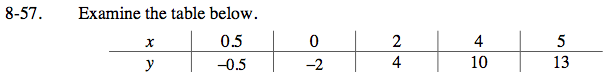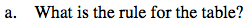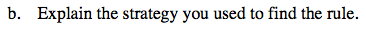Home > ACC7 > Chapter cc38 > Lesson cc38.2.1 > Problem8-57

8-57.
1. Examine the table below. Homework Help ✎

2.  x 0.5 0 2 4 5 y –0.5 –2 4 10 13
1. What is the rule for the table?

2. Explain the strategy you used to find the rule.What is y when x = 0?
What is the growth?

y = 3x − 2Based on x = 0, did you determine that −2 is a part of the rule? What else did you do?